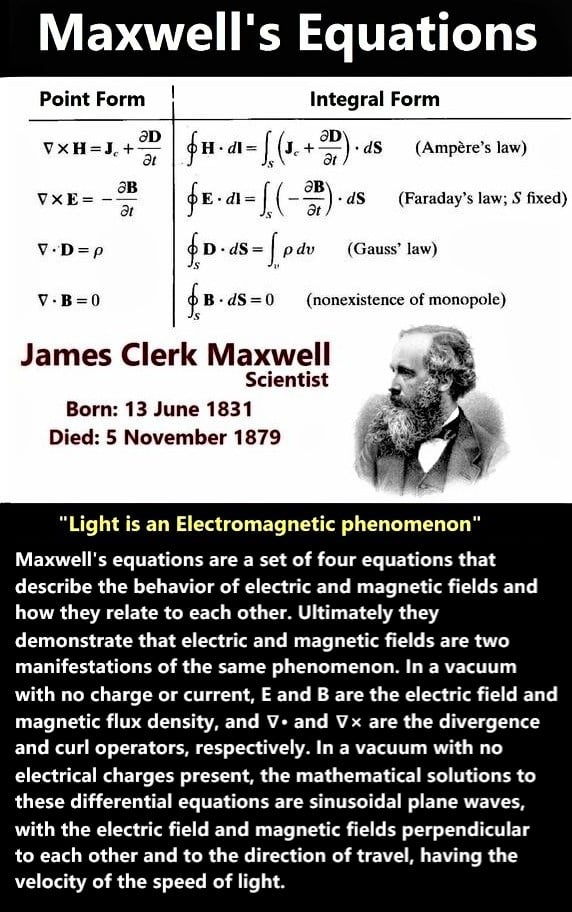# Maxwell Equations

Maxwell's equations are a set of partial differential equations that, together with the Lorentz force law, form the foundation of classical electrodynamics, classical optics, and electric circuits. These in turn underlie modern electrical and communications technologies.

Maxwell's equations have two major variants. The "microscopic" set of Maxwell's equations uses total charge and total current including the difficult-to-calculate atomic level charges and currents in materials. The "macroscopic" set of Maxwell's equations defines two new auxiliary fields that can sidestep having to know these 'atomic' sized charges and currents."Not a single one of the equations universally taught as "Maxwell's equations" ever appeared in any book or paper by James Clerk Maxwell; instead, they are Oliver Heaviside's equations. Maxwell's actual theory was written in quaternions, which is a complete system of mathematics." [Bearden, The Final Secret of Free Energy]

ECE theory demonstrates that there are additional terms to be added to standard Maxwell Heaviside equations of conventional EM theory. These terms are the scalar and vector spin connection, ω0 and ω.
These terms can be considered as the time component of an axis of rotation and the space components of rotation respectively.
So, now the updated equation E = -(∇ + ω)Φ replaces the standard E = -∇Φ where Φ is the electric potential.
This added spin connection, ω allows electricity to be directly drawn from space as explained at the above mentioned websites.